#Function Repository Resource:

# PhaseUnwrap

Remove phase jumps from phase angle data

Contributed by: Sander Huisman  |  SHuisman
 ResourceFunction["PhaseUnwrap"][list] removes phase jumps in consecutive elements of list by adding ±n 2π for jumps that are larger than the default tolerance of π. ResourceFunction["PhaseUnwrap"][list,τ] removes phase jumps in consecutive elements of list by adding ±n τ for jumps that are larger than the default tolerance of τ/2. ResourceFunction["PhaseUnwrap"][list,τ,tol] removes phase jumps in consecutive elements of list by adding ±n τ for jumps that are larger than the tolerance tol.

## Details and Options

list should be a one-dimensional numerical list.
The default for τ is 2π.
Common settings for τ are 2π and 360 for the use of angles measured in radians and degrees, respectively.
The default tolerance tol is τ/2.
A tolerance tol smaller than τ/2 works effectively the same as a tolerance of τ/2.
ResourceFunction["PhaseUnwrap"] could be seen as the opposite of Mod for a time series as it "unwraps" the "wrapping around" in modular arithmetic.
Possible forms for tol are:
 α uses the tolerance α Scaled[α] uses a tolerance of α τ

## Examples

### Basic Examples (2)

Remove the discontinuity in the phase:

 In:=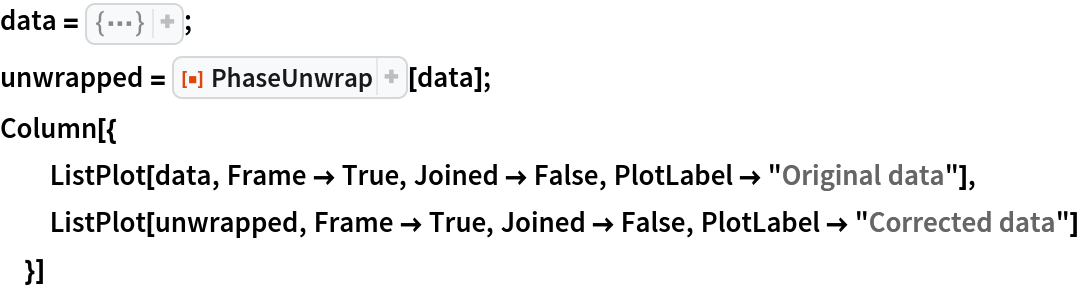Out=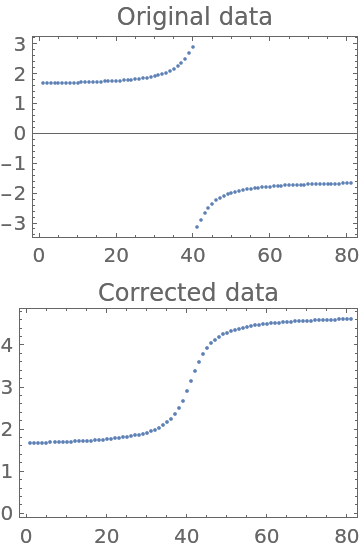Correct phase jumps in a list of phase angles measured in degrees:

 In:=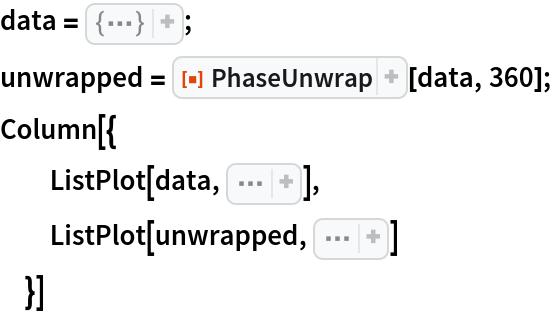Out=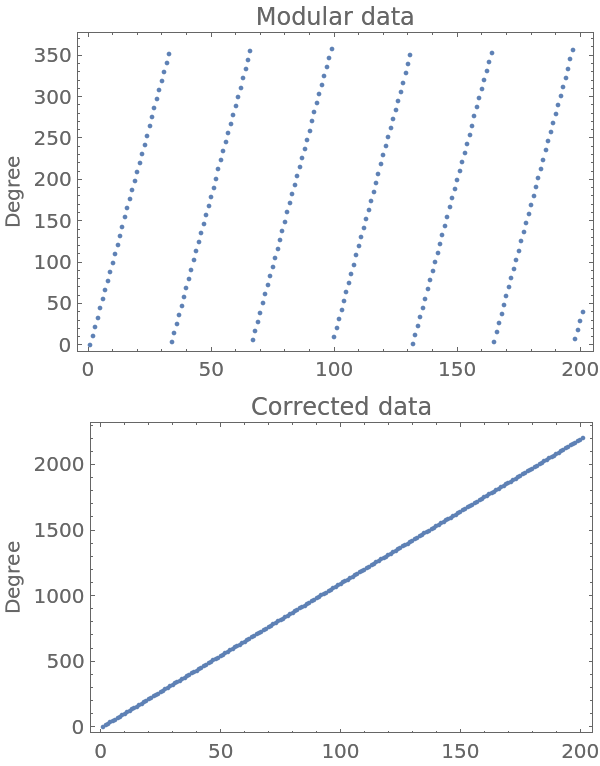### Scope (3)

Supply a stricter tolerance:

 In:=Out=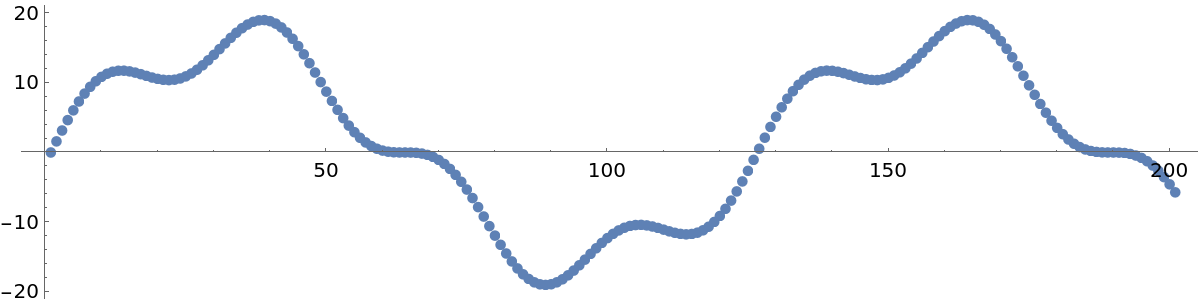Supply a tolerance as a scaled version of the modulus:

 In:=Out=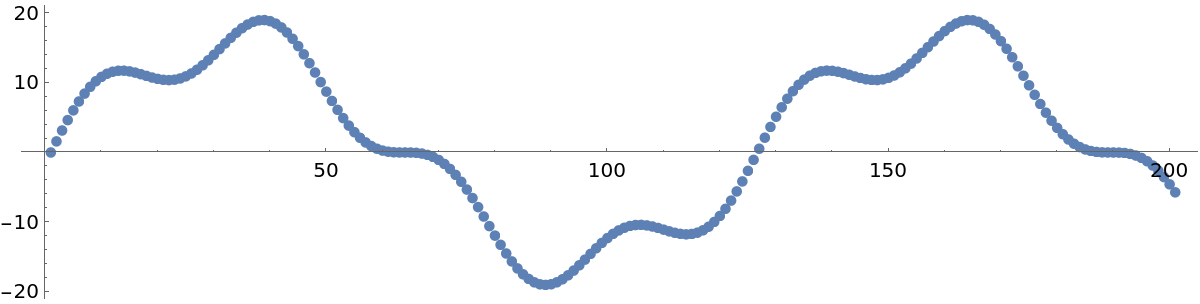Jumps of multiple times the modulus τ are also removed:

 In:=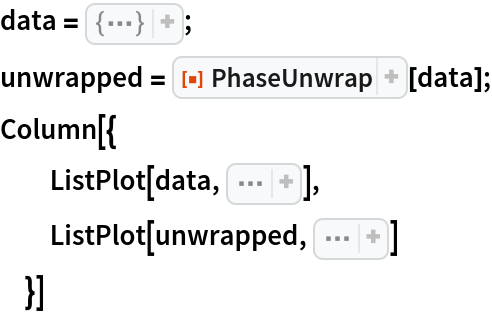Out=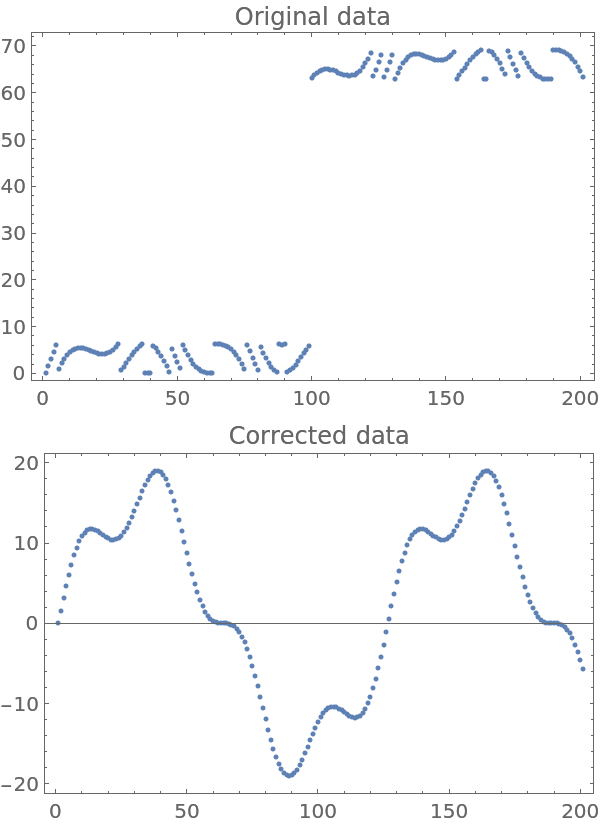### Applications (2)

Data measured from an angular encoder on a shaft:

 In:=Out=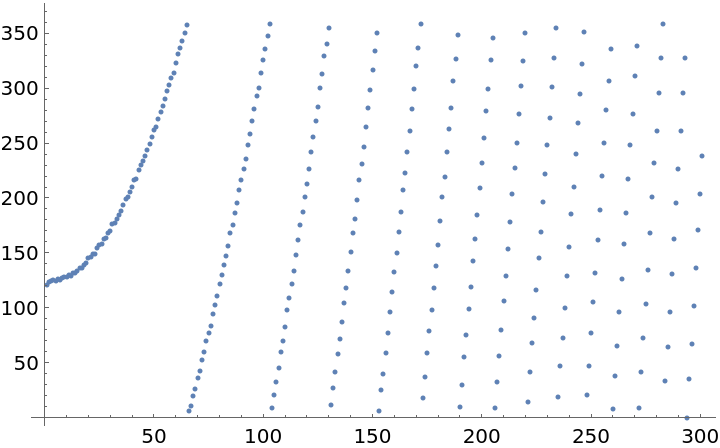Unwrap data to get the absolute angle of the shaft:

 In:=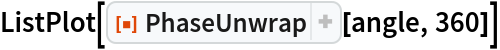Out=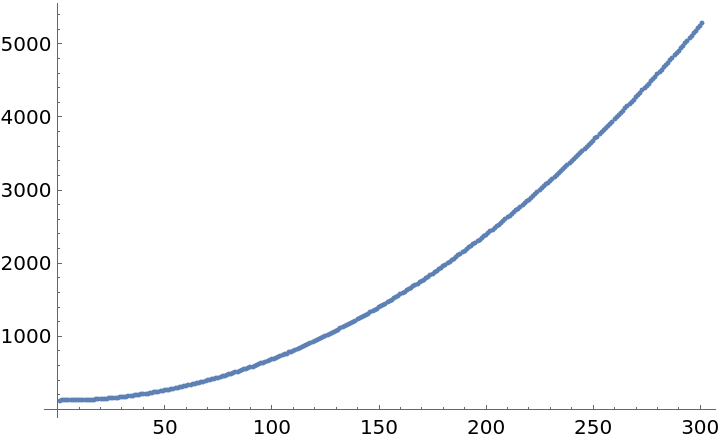### Properties and Relations (1)

PhaseUnwrap is the opposite of Mod for time series of data:

 In:=Out=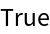### Possible Issues (1)

If the tolerance is too high, steep parts of the function cannot be reconstructed, resulting in partial removal of the discontinuities:

 In:=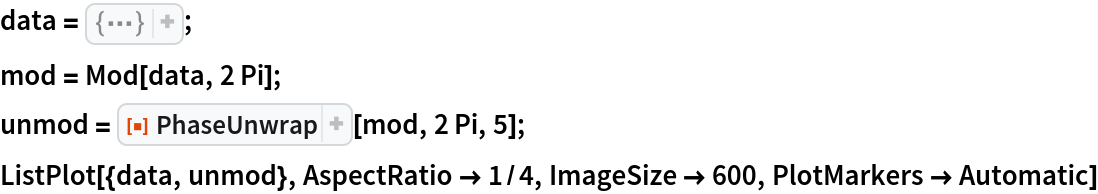Out=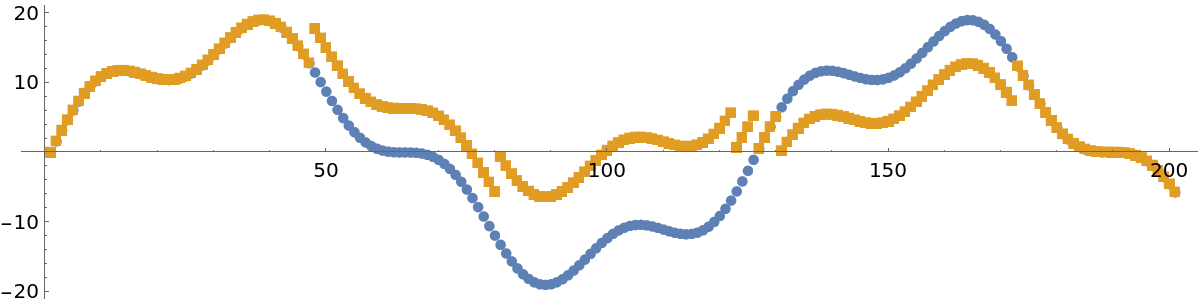### Neat Examples (2)

Show some modulated data:

 In:=Out=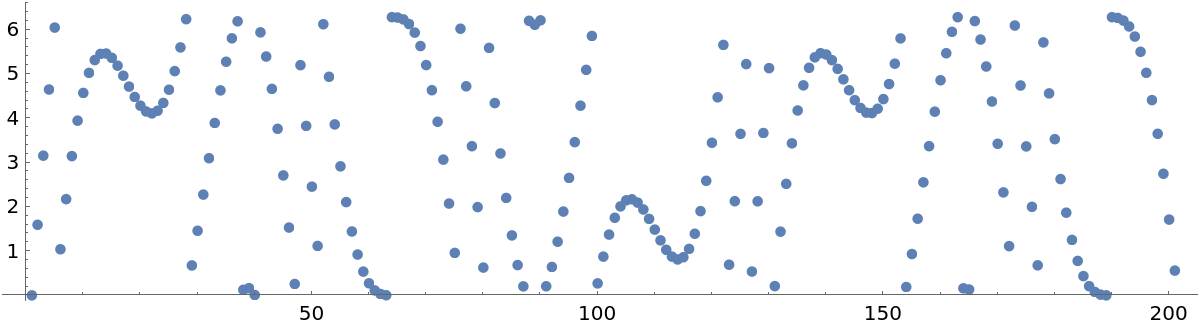Interactively change the tolerance. For a scaled tolerance of 1, the original signal is returned:

 In:=Out=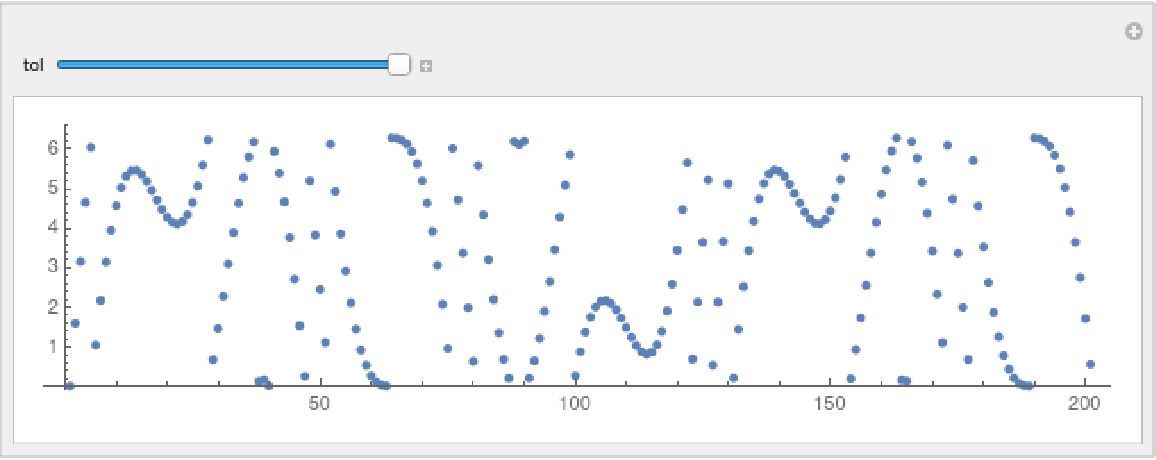Apply it to two-dimensional data by doing row-by-row unwrapping. First create some data:

 In:=Out=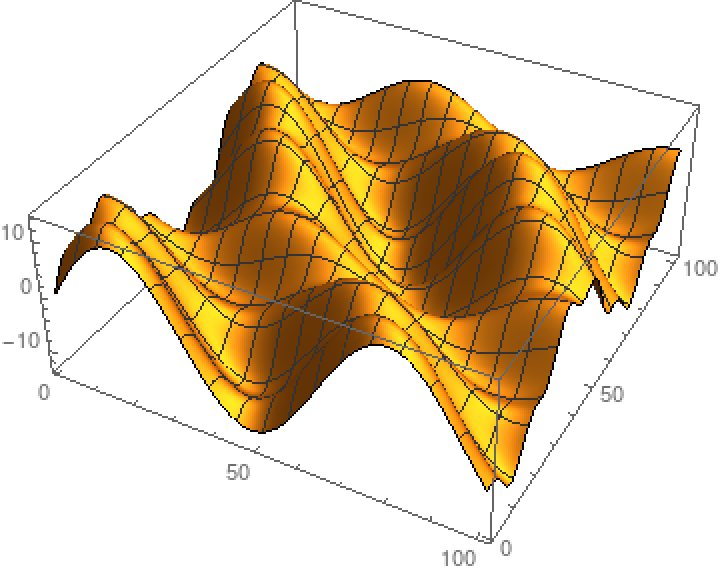Modulate the data:

 In:=Out=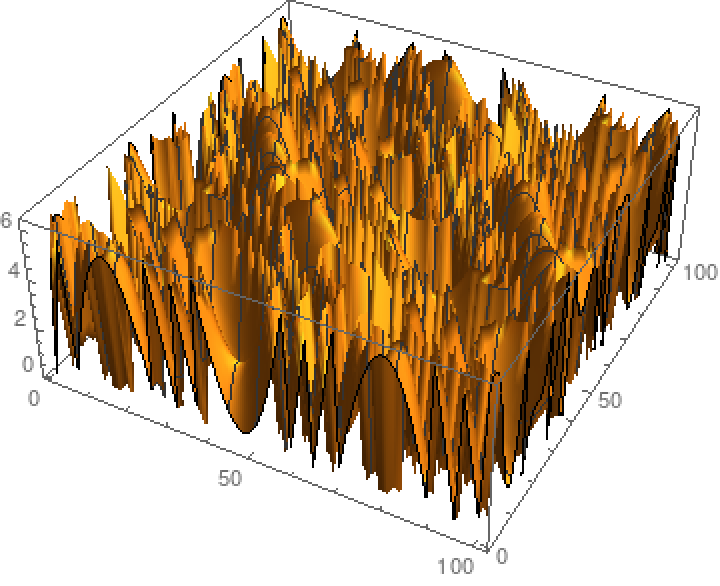Reconstruct the data:

 In:=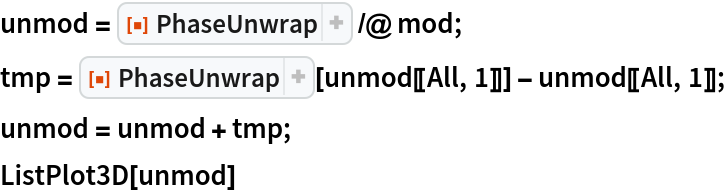Out=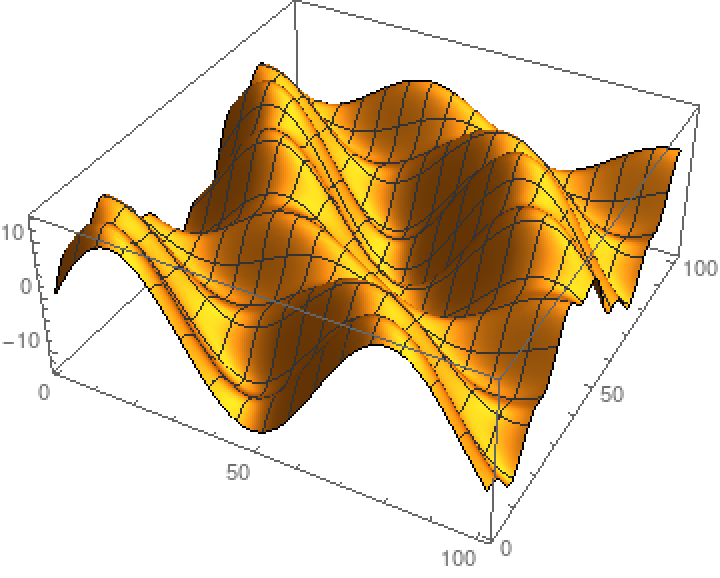Check that they are the same within machine precision:

 In:=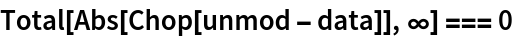Out=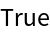## Requirements

Wolfram Language 11.3 (March 2018) or above